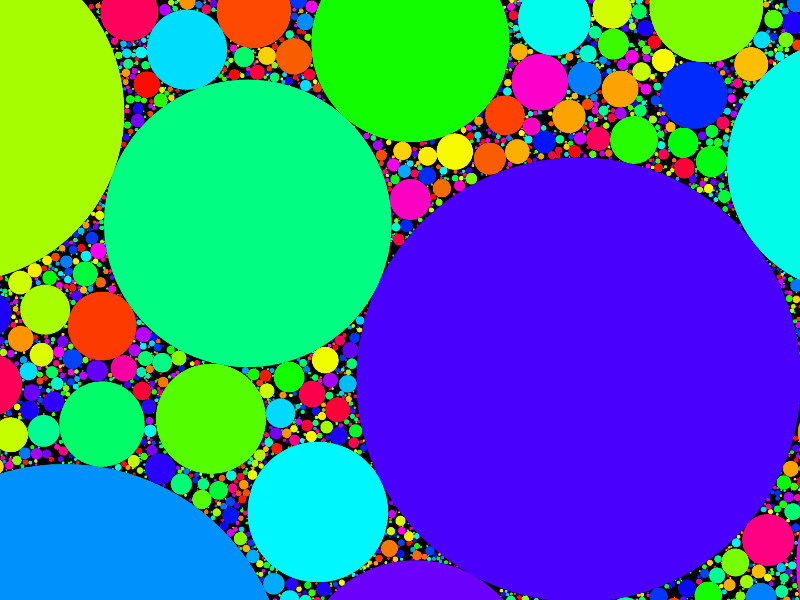• Savehttps://www.dwitter.net/d/15016

Javascript code...

r=_=>Math.random()*2e3;X=r(Y=r(Z=400));t?A.map(a=>Z=(R=((a.X-X)**2+(a.Y-Y)**2)**.5)>a.Z+Z?Z:R-a.Z):A=[];with(x)Z>0&&beginPath(A.push({X,Y,Z}),fillStyle=`hsl(\${r()} 99%50%`,fill(arc(X,Y,Z,0,7)));

426 views

keyboard shortcuts: L or F like